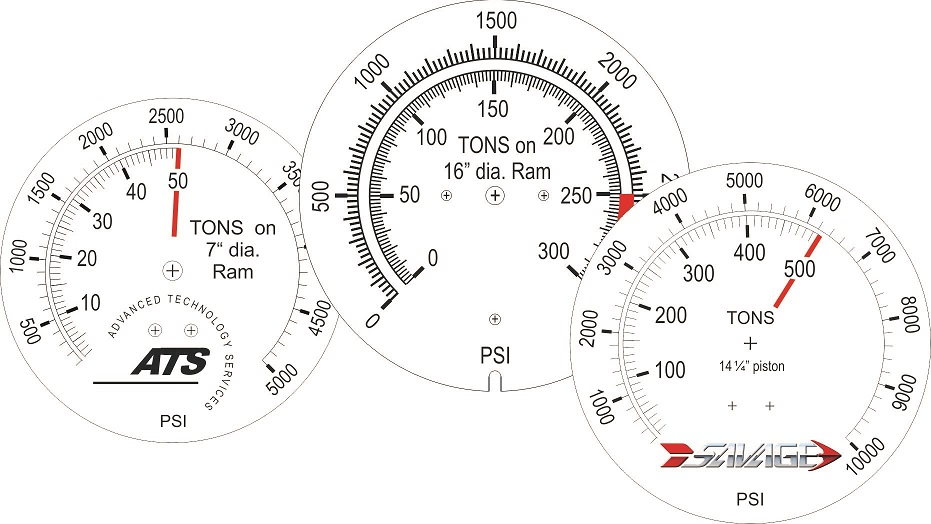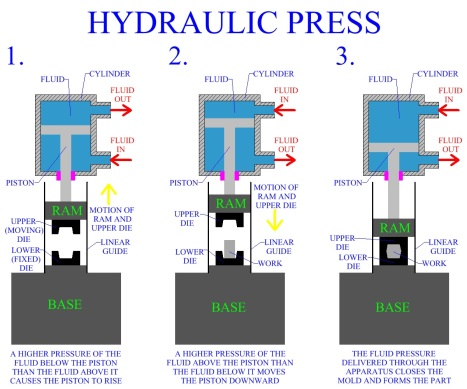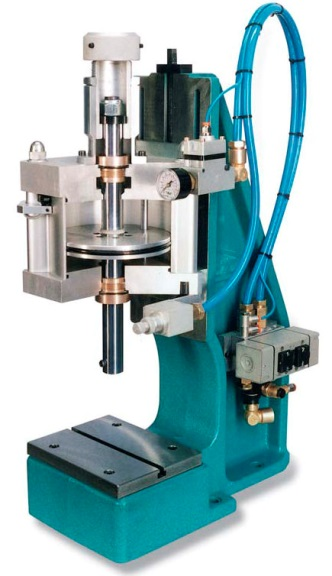# Need to understand Tons on Ram or Force Pressure Gauges? We have you covered.

While there are many stocked gauges that may meet your needs, sometimes, a custom gauge is needed for a Force Pressure Gauge.  Let's explore those Force Pressure Gauges for a moment....Pressure gauges, by design, measure pressure.  Pressure, by definition is force applied over an area.  A Ton is equivalent to 2000 pounds force.

Perry’s Engineering Handbook tells us P (Pressure) = F (Force) / A (Area).  One example is Lbsf /in2 which is PSI. However, if we put a gauge on a specific hydraulic (oil driven) or pneumatic (air driven) press, we might like to know how much FORCE is being applied between the platens.  If we know the area of the piston we can solve the above equation like this:  F = P x A.  So if the piston (aka Ram) has an area of 18 in2 and we put 1000 PSI (lbs/in2) on top of the piston it will exert (1000 x 18) or 18000 Lbs ( 9 tons) of force to the platens. (The force scale is only applicable to that specific area of piston.) If this is normal operating pressure then a custom dial might be necessary.

For safety and longevity of the equipment, the dial should display twice the expected range.  For our example, we would want a custom dial that displayed BOTH 0-2,000 PSI and 0-18 Tons on 18 sq in Ram.  If your operator needs to read in pounds, then the dial can read 0-36000 pounds force, instead of tons.  Either way, that gauge is now married to that press.

Most U.S. made presses use round pistons with standard English system diameters, usually measured to ¼”.  So there might be an 8¼” Ram for instance, or a 10” ram.  Since a circle has an area of πr2 where r is the radius (diameter/2), the area of a piston is not generally as easy as our 18 sq in Ram above.  A 6” diameter ram has an area of 3.14159 (π) x 3 x 3 or 28.274 sq. in.  So 1000 PSI on that piston would generate 28274 pounds of force or just over 14 Tons.   Obviously, it's important for your dial to be accurate.